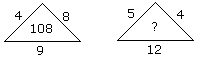# Verbal Reasoning - Character Puzzles - Discussion

### Discussion :: Character Puzzles - Character Puzzles 1 (Q.No.52)

52.

Which one will replace the question mark ?[A]. 80 [B]. 114 [C]. 108 [D]. None of these

Explanation:

(4 + 8) x 9 = 108

(5 + 4) x 12 = 108.

 Biplab said: (Jul 3, 2019) Please explain in detail.

 Avinash Rsp said: (Nov 8, 2020) (4*9) + (8*9) = 36+72 = 108. (5*12) + (4*12) = 60+48 = 108.